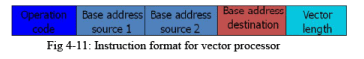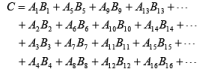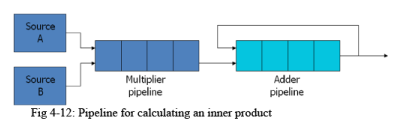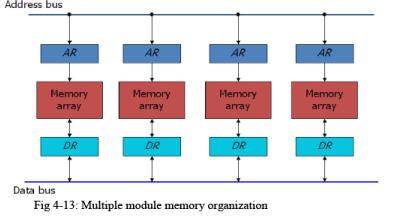Courses

# Vector Processing Computer Science Engineering (CSE) Notes | EduRev

## Electronics and Communication Engineering (ECE) : Vector Processing Computer Science Engineering (CSE) Notes | EduRev

The document Vector Processing Computer Science Engineering (CSE) Notes | EduRev is a part of the Electronics and Communication Engineering (ECE) Course Digital Electronics.
All you need of Electronics and Communication Engineering (ECE) at this link: Electronics and Communication Engineering (ECE)

Vector Processing

• In many science and engineering applications, the problems can be formulated in terms of vectors and matrices that lend themselves to vector processing. ·
• Computers with vector processing capabilities are in demand in specialized applications. e.g.
• Long-range weather forecasting
• Petroleum explorations
• Seismic data analysis
• Medical diagnosis
• Artificial intelligence and expert systems
• Image processing
• Mapping the human genome
• To achieve the required level of high performance it is necessary to utilize the fastest and most reliable hardware and apply innovative procedures from vector and parallel processing techniques.

Vector Operations

• Many scientific problems require arithmetic operations on large arrays of numbers.
• A vector is an ordered set of a one-dimensional array of data items.
• A vector V of length n is represented as a row vector by V=[v1,v2,…,Vn].
• To examine the difference between a conventional scalar processor and a vector processor, consider the following Fortran DO loop:

DO 20 I = 1, 100
20      C(I) = B(I) + A(I)

• This is implemented in machine language by the following sequence of operations.

Initialize I=0
Store C(I) = A(I)+B(I)
Increment I = I + 1
If I <= 100 go to 20
Continue

• A computer capable of vector processing eliminates the overhead associated with the time it takes to fetch and execute the instructions in the program loop.
C(1:100) = A(1:100) + B(1:100)
• A possible instruction format for a vector instruction is shown in Fig. 4-11.
• This assumes that the vector operands reside in memory.
• It is also possible to design the processor with a large number of registers and store all operands in registers prior to the addition operation.
• The base address and length in the vector instruction specify a group of CPU registers.Matrix Multiplication

• The multiplication of two n x n matrices consists of n2 inner products or n3 multiply-add operations.
• Consider, for example, the multiplication of two 3 x 3 matrices A and B.
• c11= a11b11+ a12b21+ a13b31
• This requires three multiplication and (after initializing c11 to 0) three additions.
• In general, the inner product consists of the sum of k product terms of the form
C= A1B1+A2B2+A3B3+…+AkBk.
• In a typical application k may be equal to 100 or even 1000.
• The inner product calculation on a pipeline vector processor is shown in Fig. 4-12.Memory Interleaving

• Pipeline and vector processors often require simultaneous access to memory from two or more sources.
• An instruction pipeline may require the fetching of an instruction and an operand at the same time from two different segments.
• An arithmetic pipeline usually requires two or more operands to enter the pipeline at the same time.
• Instead of using two memory buses for simultaneous access, the memory can be partitioned into a number of modules connected to a common memory address and data buses.
• A memory module is a memory array together with its own address and data registers.
• Fig. 4-13 shows a memory unit with four modules.• The advantage of a modular memory is that it allows the use of a technique called interleaving.
• In an interleaved memory, different sets of addresses are assigned to different memory modules.
• By staggering the memory access, the effective memory cycle time can be reduced by a factor close to the number of modules.

Supercomputers

• A commercial computer with vector instructions and pipelined floating-point arithmetic operations is referred to as a supercomputer.
• To speed up the operation, the components are packed tightly together to minimize the distance that the electronic signals have to travel.
• This is augmented by instructions that process vectors and combinations of scalars and vectors.
• A supercomputer is a computer system best known for its high computational speed, fast and large memory systems, and the extensive use of parallel processing.
• It is equipped with multiple functional units and each unit has its own pipeline configuration.
• It is specifically optimized for the type of numerical calculations involving vectors and matrices of floating-point numbers.
• They are limited in their use to a number of scientific applications, such as numerical weather forecasting, seismic wave analysis, and space research.
• A measure used to evaluate computers in their ability to perform a given number of floating-point operations per second is referred to as flops.
• A typical supercomputer has a basic cycle time of 4 to 20 ns.
• The examples of supercomputer:
• Cray-1: it uses vector processing with 12 distinct functional units in parallel; a large number of registers (over 150); multiprocessor configuration (Cray X-MP and Cray Y-MP)
• Fujitsu VP-200: 83 vector instructions and 195 scalar instructions; 300 megaflops
Offer running on EduRev: Apply code STAYHOME200 to get INR 200 off on our premium plan EduRev Infinity!

## Digital Electronics

92 videos|91 docs|12 tests

,

,

,

,

,

,

,

,

,

,

,

,

,

,

,

,

,

,

,

,

,

;